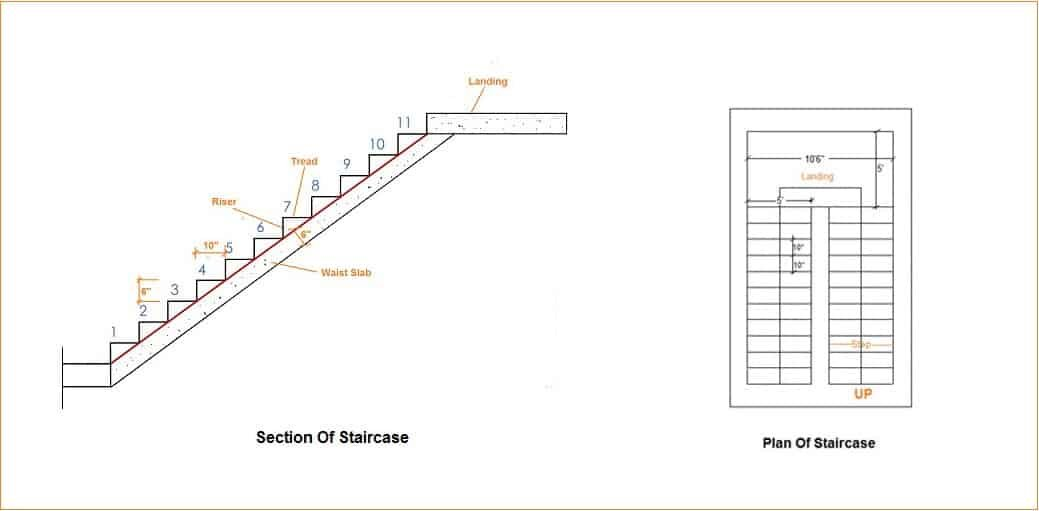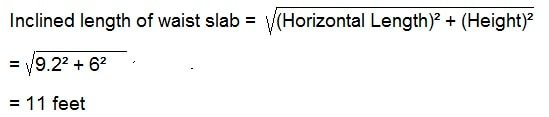# How To Calculate Volume Of Concrete For Staircase

## How To Calculate Volume Of Concrete For Staircase:

Staircase is one of the essential parts of a building. It helps people to access different floors in a building. In this article, I will explain how to estimate concrete volume and quantity of cement, sand, aggregates for a staircase. Before starting the estimation, we need to know different components of a staircase which I will use in this calculation.

## Steps:

A portion of stairway comprising the tread and riser which permits ascent and descent from one floor to another.

The horizontal upper part of a step on which foot is placed in ascending or descending stairway.

## Riser:

The vertical portion of a step providing support to the tread.

## Flight:

A series of steps without any platform, break or landing in their direction.

## Landing:

A platform or resting place provided between two flights.

Now coming to the calculation of concrete volume for the staircase.

Let’s take an example:The height of one floor = 12 feet.

Height to be acquired by one flight = 12/2 = 6 feet.

READ -  How To Calculate Quantity Of Soil/Sand For Filling Plot

Risers = 6″ = 0.5 feet.

Number of risers = Height of flight / Riser = 6/0.5 = 12

Tread = 10″ = 0.8 feet.

Number of treads = (Numbers of risers -1) = 12 – 1

Thickness of waist slab = 6″ = 0.5 feet

Length of step = 5 feet

## Volume Of Concrete For Steps:

Volume of one step = 1/2 x riser x tread x length of step

= 1/2 x 0.5 x 0.8 x 5 = 1.03 = 1 cubic feet.

As we have 11 number of steps in a flight the volume of steps for first flight  = 11 x 1 = 11 cubic feet.

## Concrete Volume For Waist Slab:

To calculate the volume of concrete for waist slab we need to know the inclined length of the waist slab.

Horizontal length of waist slab = Tread x Number of steps

=0.8333′ x 11 = 9.2 feet.

The height of the top of the landing from floor = Number of riser x Height of riser

= 12 x 0.5 = 6 feet.The volume of concrete for waist slab = Inclined length of waist slab x Width of waist slab x Thickness of waist slab

= 11′ x 5′ x 0.5′

= 27.5 cubic feet

∴ Volume of concrete for first flight = Volume of waist slab + Volume of steps

= 27.5+11 = 38.5 cubic feet

## Volume Of Concrete For Landing Of Staircase:

Length of landing = 10.5 feet

Width of landing = 5 feet

Thickness of landing = 0.5 feet

∴ Concrete volume for landing = 10.5′ x 5′ x 0.5′ =  26 cubic feet.

## Concrete Volume for 2nd Flight Of The Staircase:

As the 1st light and 2nd flight are same in our staircase so the volume of concrete will be same.

Concrete volume for 2nd flight of the staircase = 38.5 cubic feet.

Total volume of concrete for staircase = Volume of first flight + Volume of second flight + Volume of landing

= 38.5 + 38.5 + 26

= 103 cubic feet.

Well, this is the wet volume of concrete. To convert wet volume into dry volume we need to multiply with 1.54

Dry volume of concrete = 103 x 1.54 = 159 cubic feet.

## Quantity Of Materials For Staircase:

We will calculate for 1:2:4 Concrete mix.

1. Volume of cement = 1/7 x Dry volume = 1/7 x 159 = 22.66 cubic feet.

Number of Cement bags = volume of cement / Volume of cement bag  = 22.66 / 1.25 = 18 No’s of bag. (The volume of one bag cement of 50 kg = 1.25 cubic feet)

2. Volume of Sand = 2/7 x Dry volume = 2/7 x 159 = 45 cubic feet.

3. Volume of Aggregate = 4/7 x Dry volume = 4/7 x 159 = 91 cubic feet.

Note: Here I have used cubic feet unit and 1:2:4 mix ratio, you can use cubic meter unit and any other mix ratio of concrete.